# CS21 Lab 10: Recursion

Due 11:59pm Saturday, Apr 18, 2015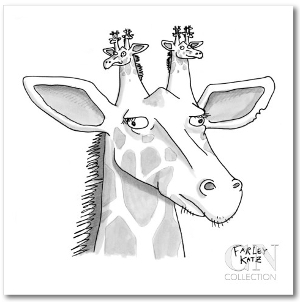Run update21, if you haven't already, to create the cs21/labs/10 directory. Then cd into your cs21/labs/10 directory and create the python programs for lab 10 in this directory.

Recursion

This week we will write a few smaller programs, all using recursion.

Some of the programs will use the Zelle graphics library again.

1. Silly Text

Write a program to create a silly text effect in the terminal window. Your program should (in main()) ask the user for some text, then ask for a character. After getting the user's input, call a recursive function that creates and returns a string, where each letter of the original text is followed by a certain number of the input character. As shown below, the number of characters between each letter decreases with each letter.

Your recursive function should have just 2 parameters: the text string and the input character that goes between the letters. The function should return the final string to be printed in main().

```\$ python sillytext.py

string: Swarthmore
pattern: .

S.........w........a.......r......t.....h....m...o..r.e

\$ python sillytext.py

string: 12345
pattern: *

1****2***3**4*5
```

2. Ruler

Write a program called ruler.py that displays simple ruler tick marks to the terminal window. The number of tick marks printed on each line follows a pattern as shown below. Your program should have a main function that asks the user for n, then calls a recursive function to print the dashes.

```\$ python ruler.py

n: 2

-
--
-

\$ python ruler.py

n: 3

-
--
-
---
-
--
-

\$ python ruler.py

n: 4

-
--
-
---
-
--
-
----
-
--
-
---
-
--
-

```

Hint: notice the n=3 case is made up of the n=2 case, followed by 3 dashes, and then the n=2 case again. Same for the n=4 case (just n=3, 4 dashes, then n=3 again).

3. Recursive Graphics

Write a graphics program that uses recursion to draw a picture.

First write a graphics function to draw an object, like a star, a flower, a smiley face, or anything you choose. However, your function must work with the following parameters:

• a graphics window object
• a center point (where to draw your object)
• a size (how big to draw your object)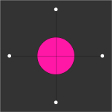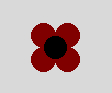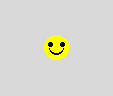Now write a full program called recursive-graphics.py that draws a picture similar to those below. Your main function should set up the graphics window and then call a recursive function to draw the objects. Your recursive function, when it is ready to draw one of the objects, should call your original drawStar() function (or drawFlower(), etc).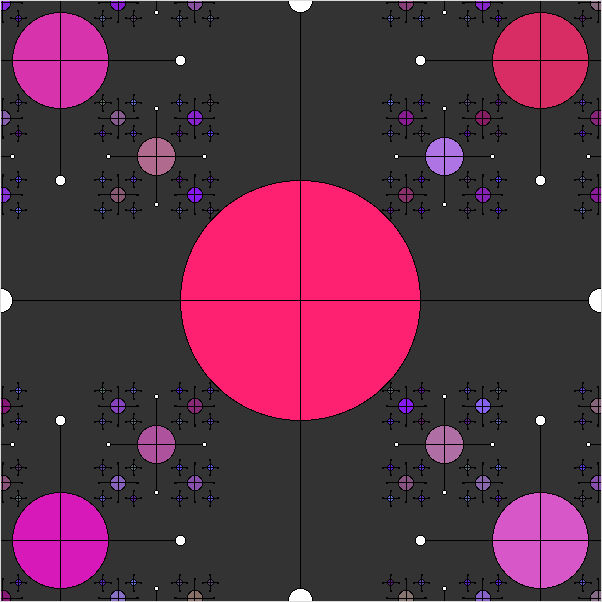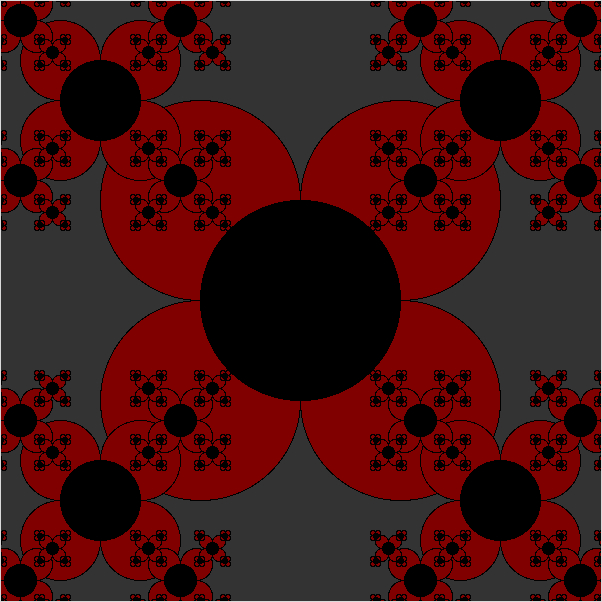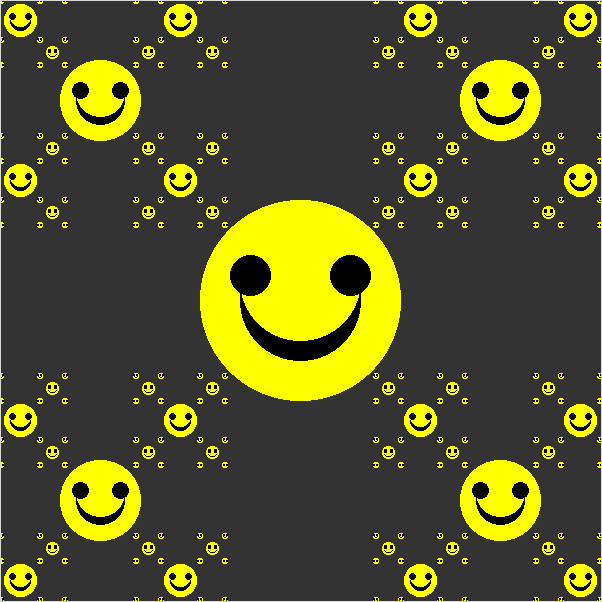4. Verner Panton

Write a graphics program called panton.py that uses recursion to create images similar to these Verner Panton textile patterns. Here are some possible images created by our program: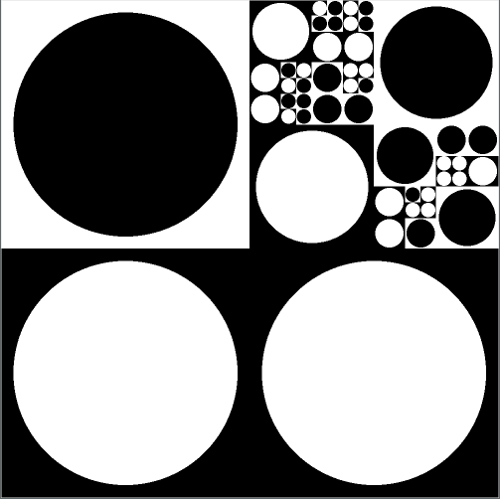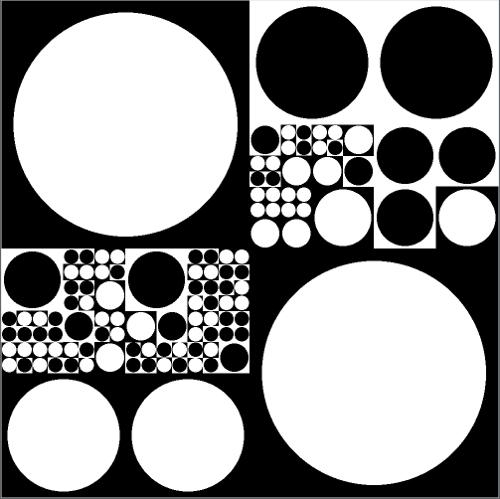For this program, you should again have a main function that sets up the graphics window and then calls a recursive function to draw the squares and circles. Your recursive function should have 4 parameters: the GraphWin object, the upper left and lower right Point objects, and a size. The recursive function should include some randomness, to decide if it should draw the given square (using the upper left and lower right points) and circle (using the size as the radius), or subdivide the given square into 4 equal squares and recur (assuming the current size is large enough to allow subdividing). You should also use random library functions to decide what to draw: black squares with white circles, or white squares with black circles.

• use clone() to make copies of Point objects:
```  p1 = Point(100,200)
p2 = p1.clone()
p2.move(100,0)
```
• the Rectangle(p1,p2) constructor works with either the upperleft/lowerright points or the lowerleft/upperright points

Hacker's Challenges
These are just fun, optional additions. Try these if you are looking for more to do (no bonus points).
1. Write a program called graphicsruler.py that implements a similar recursive function using the graphics library:
```\$ python graphicsruler.py
n: 5
```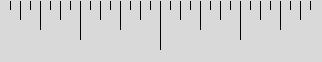2. Write a program called color-panton.py that uses HSV color values to create a rainbow effect for the textile pattern: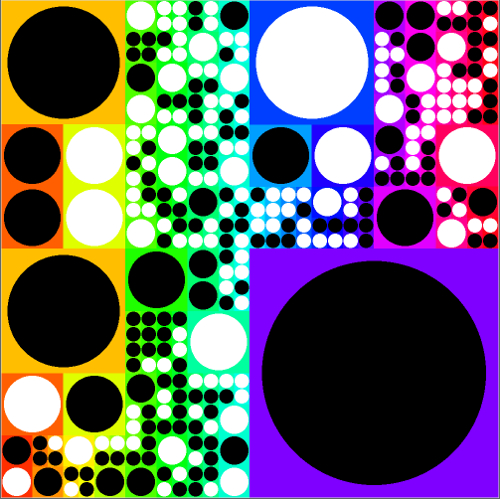Hint: the color of the square is tied to the X axis position, so a square at X=0 would have a hue value of 0, and a square at X=width would have a hue value of 1.0.

You can convert from HSV to RGB values using the following:

```import colorsys

hue = 0.5  # could be any number from 0 to 1
r,g,b = colorsys.hsv_to_rgb(hue, 1.0, 1.0)
red = int(r*255)
green = int(g*255)
blue = int(b*255)
color = color_rgb(red, green, blue)
# then use color variable as fill for square
```

Submit

Once you are satisfied with your program, hand it in by typing handin21 in a terminal window.